# Homepage of KerMet-Tools

## Content

Bernard Haasdonkmathematik.uni-stuttgart.de

```KerMet-Tools V1.2                                           March 16, 2009
-------------------------------------------------------------------------
Kernel-Method MATLAB Tools

This toolbox provides MATLAB routines for pattern analysis with
kernel methods. Excellent textbooks on this topic comprise
[Sc:Sm:02], [Sh:Cr:04] or [He:02]. The implemented kernel methods include
SVM, KPCA, kernel nearest-neighbour, kernel perceptron, enclosing
hypersphere algorithm, kernel fisher discriminant and kernel-nearest
mean classifier.

Additionally it provides different kernel functions which incorporate
invariance knowledge as presented in [Ha:Vo:Bu:05], [Ha:Ke:02] and [Ha:Bu:07].
The use of the functions is exemplified by the main demo KerMet_Toy.

This is the release of KerMet-Tools version 1.2 on March 4, 2009.
The toolbox was tested on Linux and Windows XP to 10 using
MATLAB versions R2007a up to R2016b.

-------------------------------------------------------------------------
This library is free software; you can redistribute it and/or modify
the Free Software Foundation; either version 2 of the License, or
(at your option) any later version.

This library is distributed in the hope that it will be useful,
but WITHOUT ANY WARRANTY; without even the implied warranty of
MERCHANTABILITY or FITNESS FOR A PARTICULAR PURPOSE.  See the
GNU General Public License for more details.

You should have received a copy of the GNU General Public License
along with this program; if not, write to the Free Software
Foundation, Inc., 51 Franklin St, Fifth Floor, Boston, MA  02110-1301  USA
-------------------------------------------------------------------------

Contents:
---------
Organizational:
BUGLIST.txt         : list of known bugs

MATLAB distance matrices:
EDsq_matrix.m       : Euclidean distance matrix
IDsq_matrix.m       : Regularized invariant distance matrix, cf. [Ha:Bu:07]
TDsq_matrix.m       : Tangent distance matrix, cf. [Ha:Ke:02]

MATLAB kernel matrices:
rbf_kernel_matrix.m              : Gaussian rbf kernel matrix (pd)
tria_kernel_matrix.m             : triangular kernel kernel matrix (cpd)
linear_kernel_matrix.m           : linear kernel matrix (pd)
poly_kernel_matrix.m             : polynomial kernel matrix (pd)
pseudo_euclidean_kernel_matrix.m : pseudo-Euclidean inner product(indefinite)
rbf_DS_kernel_matrix.m           : Gaussian rbf Distance Substitution matrix
cf. [Ha:Ba:Bu:04]
tria_DS_kernel_matrix.m          : triangular kernel Distance Substitution
cf. [Ha:Ba:Bu:04]
linear_DS_kernel_matrix.m        : linear kernel Distance Substitution
cf. [Ha:Ba:Bu:04]
poly_DS_kernel_matrix.m          : polynomial kernel Distance Substitution
cf. [Ha:Ba:Bu:04]
HI_kernel_matrix.m               : Haar-Integration kernel matrix
cf. [Ha:Vo:Bu:05]
virtual_samples_kernel_matrix.m  : kernel matrix of multiplied dataset

MATLAB kernel methods:
predict_kernel_nearest_neighbour.m : prediction of kernel k-nearest-neighbour
train_two_class_C_SVM.m,
predict_two_class_C_SVM.m          : training and prediction of binary SVM
with C-penalty parameter
train_kernel_PCA.m,
predict_kernel_PCA.m               : principal component decomposition and
projection in kernel space
train_kernel_perceptron.m,
predict_kernel_perceptron.m        : training and prediction with the kernel
perceptron, cf. [He:02]
train_nu_hypersphere.m,
predict_nu_hypersphere.m           : Training and prediction of the minimum
enclosing hypersphere algorithm in the
"nu" penalty parameter version
cf. [Sh:Cr:04]

train_kernel_fisher_discriminant.m,
predict_kernel_fisher_discriminant.m : Training and Prediction of the kernel
fisher discriminant,
cf. [Mi:Ra:We:Sc:Mu:99]

train_nearest_mean_classifier.m,
predict_nearest_mean_classifier.m : Training and Prediction of the
nearest mean classifier in kernel space,
cf. [Sc:Sm:02]

MATLAB demo:
KerMet_Toy.m   : function starting the kernel method demo
KerMet_Toy.fig : GUI layout of the demo
KerMet_Toy.txt : Help-text invoked and displayed by the demo

MATLAB others:
compute_TD1sq.m     : computation of one-sided tangent distance
compute_tangents.m  : computation of tangents to transformations
transform_vectors.m : transformation of patterns
normalize.m         : normalization of vectors
orthonormalize.m    : orthonormalization of vectors
arrow.m             : routine for plotting an arrow with patch-head

Detailed description of the functions can be obtained by invoking the
MATLAB help command on the single files. An illustrated guide for the
demo KerMet_Toy can be found at
http://pnp.mathematik.uni-stuttgart.de/ians/haasdonk/KerMet-Tools/

Feedback
--------
Users are requested to kindly help us by providing the feedback about errors
occured while usage. Also suggestions for modifications are very appreciated!

Contact
-------
Bernard Haasdonk, haasdonk@mathematik.uni-stuttgart.de

Current Affiliation:
Institute of Applied Analysis and Numerical Simulation
University of Stuttgart
Pfaffenwaldring 57
70569 Stuttgart
Germany

References
----------
[Ha:Ke:02] Haasdonk, B., Keysers, D., Tangent Distance Kernels for Support
Vector Machines. ICPR 2002, International Conference on Pattern Recognition,
IEEE, 2002.

[Ha:Ba:Bu:04] Haasdonk, B., Bahlmann, C. Learning with Distance Substitution
Kernels.  Pattern Recognition - Proc. of the 26th DAGM Symposium, Tübingen,
Germany, August/September 2004. Springer Berlin, 2004.

[Ha:Bu:07] Haasdonk, B. and Burkhardt, H. Invariant Kernels for Pattern
Analysis and Machine Learning. Machine Learning, 68:35-61, 2007.

[Ha:Vo:Bu:05] Haasdonk, B., Vossen, A. and Burkhardt, H., Invariance in
Kernel Methods by Haar-Integration Kernels.  SCIA 2005, Scandinavian
Conference on Image Analysis, pp. 841-851, Springer-Verlag, 2005.

[He:02] Herbrich, R. Learning Kernel Classifiers. MIT Press, 2002.

[Mi:Ra:We:Sc:Mu:99] Mika, S., Raetsch, G., Weston, J., Schoelkopf, B.
and Mueller, K.-R., Fisher discriminant analysis with kernels. In Neural
Networks for Signal Processing, pp. 41-48, 1999

[Sc:Sm:02] Schoelkopf, B. and Smola, A. Learning with Kernels: Support Vector
Machines, Regularization, Optimization and Beyond. MIT Press, 2002.

[Sh:Cr:04] Shawe-Taylor, J. and Cristianini, N. Kernel Methods for Pattern
Analysis. Cambridge University Press, 2004.
```

## Illustrated Guide to demo KerMet_Toy

```KerMet_Toy  V1.2
================

```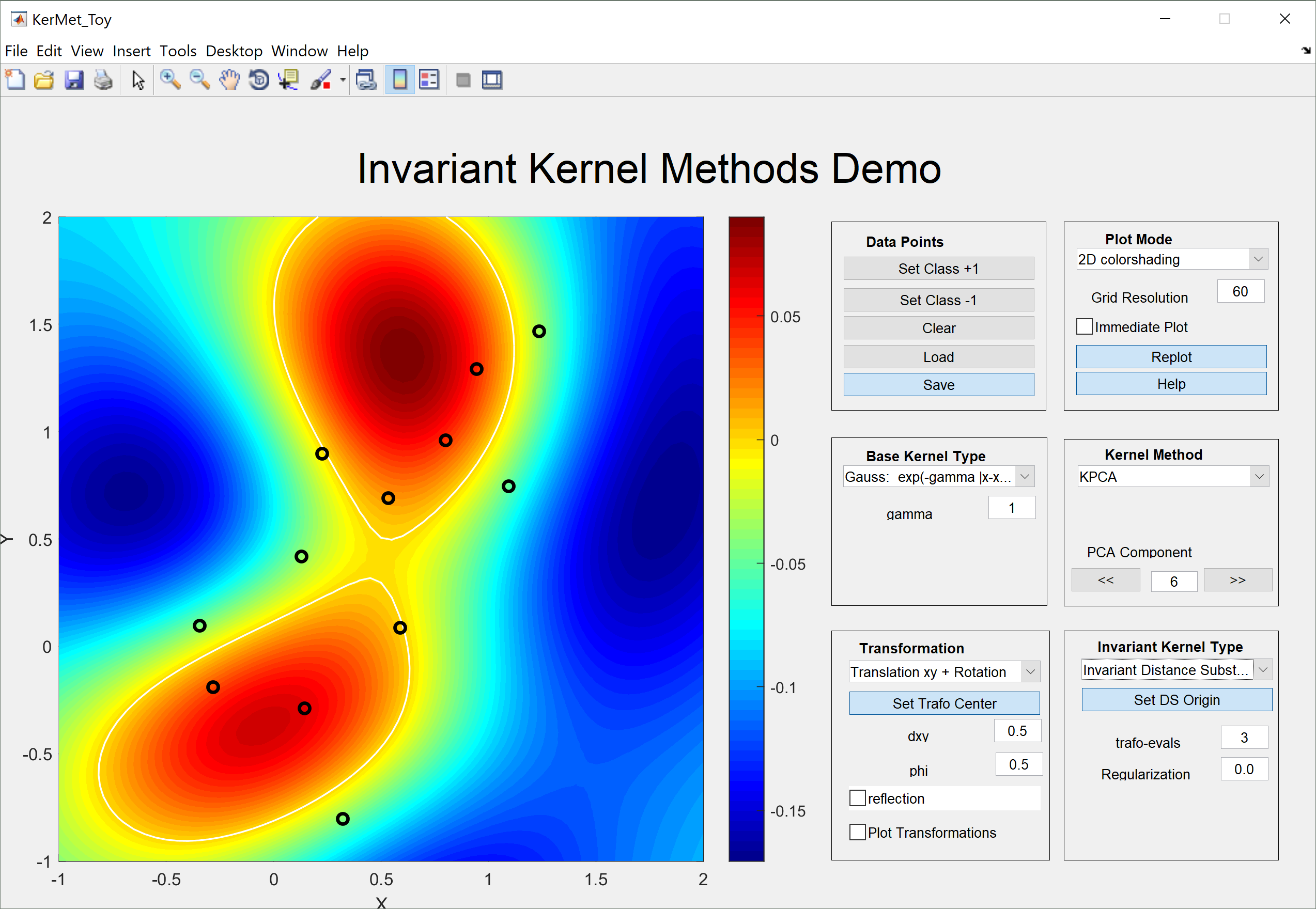```This GUI enables interactive exploration of various kernel methods, kernel
functions and invariant kernel types. The kernels, kernel-methods etc. are
encapsulated in separate m-files for use as a general "kernel method
library". Therefore, see the corresponding files for detailed help on the
components.

The main interactives in the GUI are grouped as follows:

Data Points:
------------

```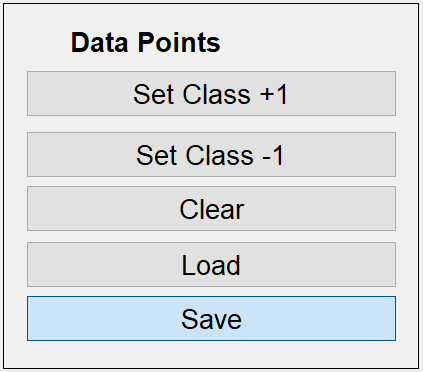```Two-class data can be set by choosing the corresponding class ("Set Class
+1","Set Class -1") and klicking points in the plot frame. Generated point
distributions can be cleared ("Clear"), stored in files ("save") and
recovered ("load"). If the kernel method does not use class information,
the points are plotted in black, otherwise blue/red discrimination is
performed.

Plot Mode:
----------

```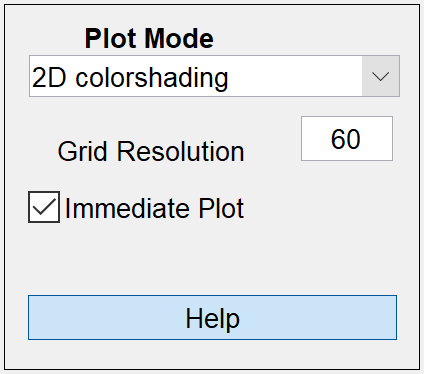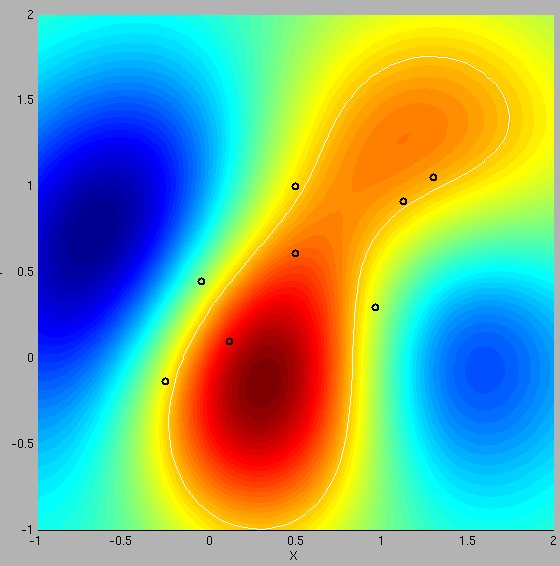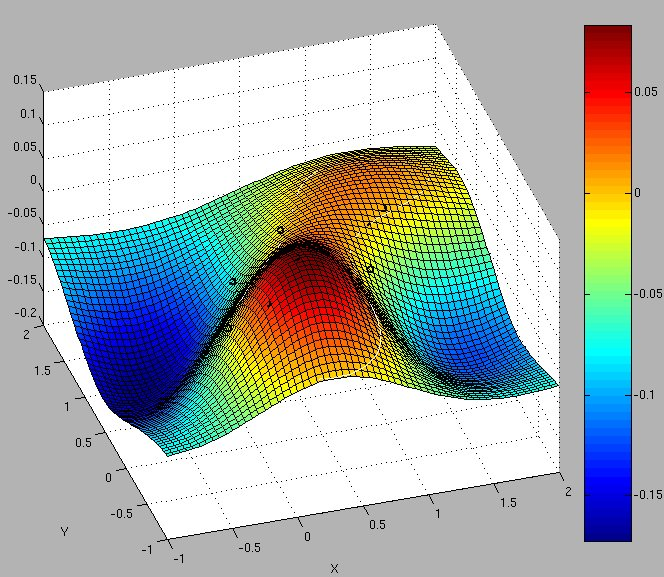```Here either 2D or 3D plot-mode can be choosen. The important parameter
"Grid Resolution" should be set adapted to the system speed. In particular
for expensive kernel methods (kernel-k-nn, KPCA) this should be lowered
during parameter tuning. Alternatively, the automatic replotting mode can
be switched off by unchecking "Immediate Plot" and pressing the appearing
"Replot" button if needed. The "Help" button displays this help text.

Base Kernel Type:
-----------------
```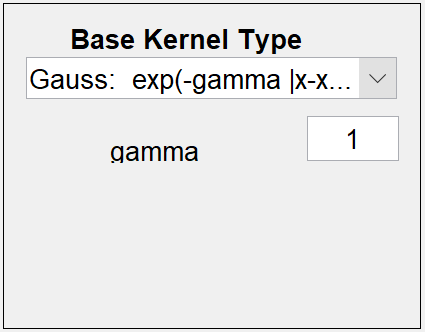```Here the base kernel and its parameters can be set. In particular these
comprise the standard linear, polynomial and Gaussian rbf-kernels. Also
non-pd kernels are included, e.g. the negative distance kernel, which is
conditionally pd (for 0 <= beta<= 2) and the pseudo-Euclidean inner
product, which is the prototype of a general indefinite inner-product.

Kernel Method:
--------------
```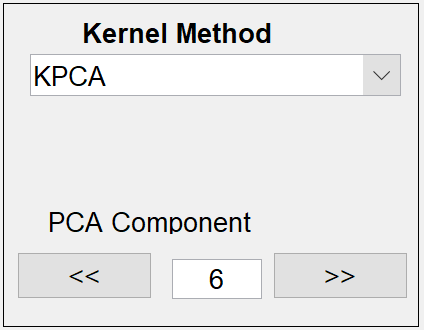```Here the kernel method and its parameters can be set. Each method mainly
relies on two functions train_* and predict_*, where * replaces the
kernel-method-name.

1. Single kernel:
```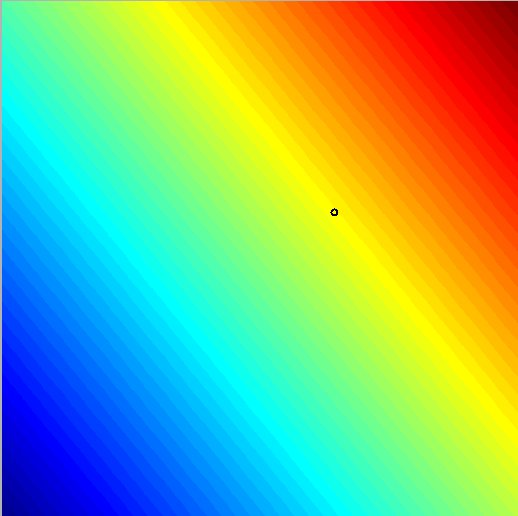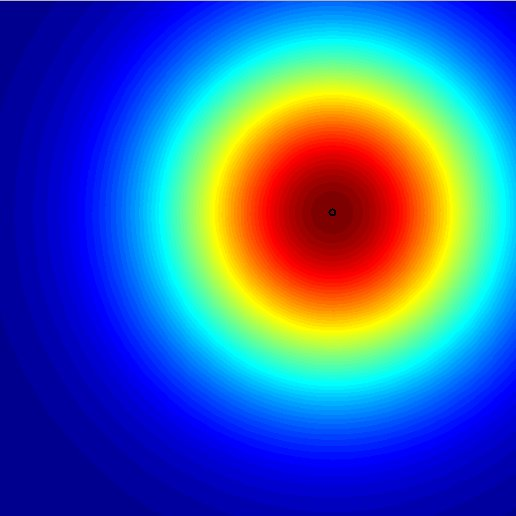```The first data point is taken as fixed argument in the specified kernel
function. The second point is varying over the 2D-plane, the kernel values
are computed and color coded. This function is very well suited for simply
visualizing single kernels and their behaviour.

2. Kernel k-nearest-neighbour:
```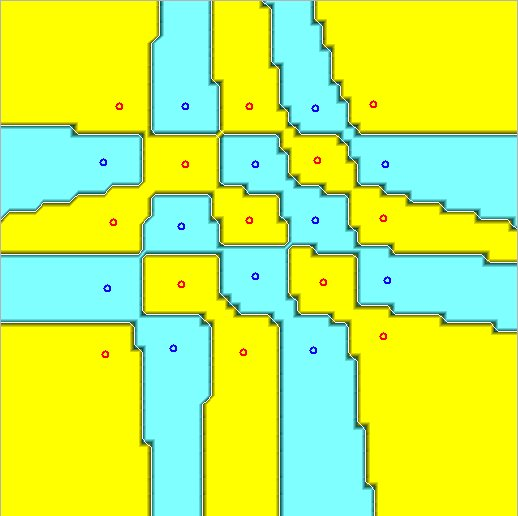```The k-nearest-neighbour algorithm for classification only relies on
distances. After defining a kernel function, the distances in the induced
feature-space can be computed from the kernel matrix. Correspondingy, the
kernelized version of distance based classifiers such as
k-nearest-neighbours can be performed reflecting certain
base-kernel-properties. The size of the neighbourhood "k" can be chosen.
The output values are the certainty, i.e. the ratio of the
class-frequencies in the k-neighbourhood of each point.

3. Support vector machine (SVM):
```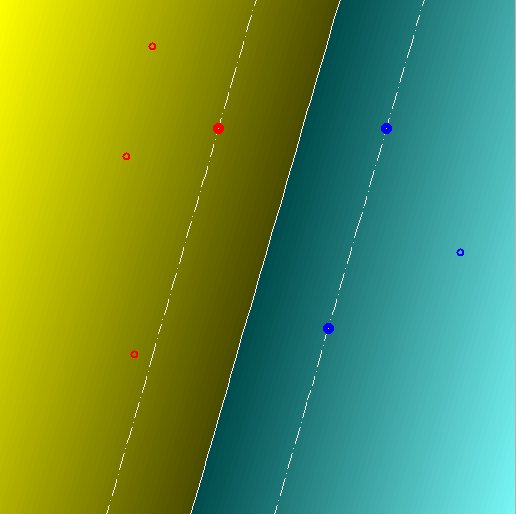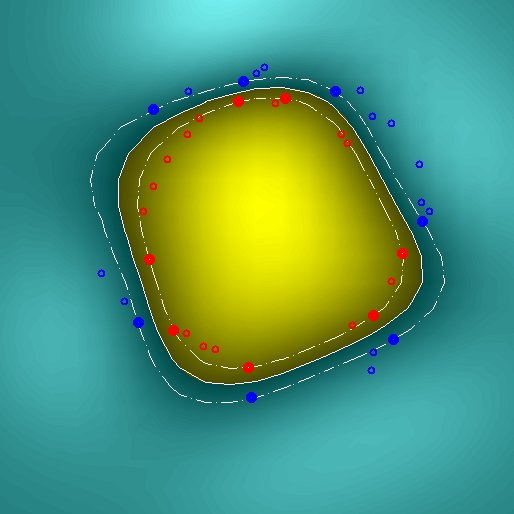```The two-class C-SVM for classification is implemented. Correspondingly,
the soft-margin penalization parameter "C" can be set. The output is the
decision value and plotting of the margin.

4. Kernel perceptron:
```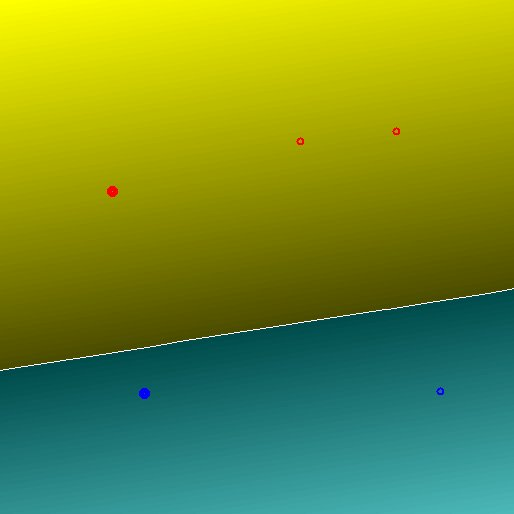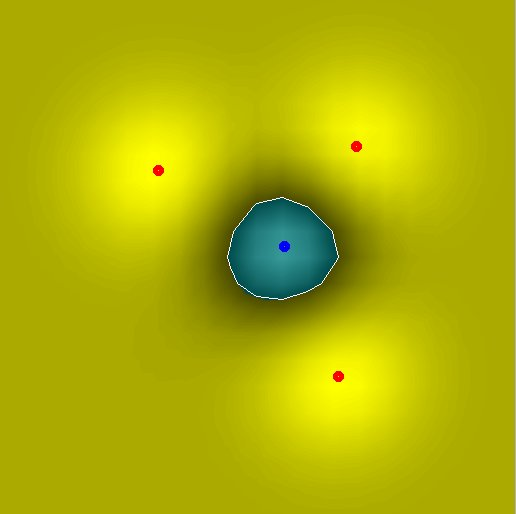```The well known perceptron for two-class classification only relies on
inner products, so a kernelized version is straightforward. The number of
update steps can be specified and the preliminary solution is plotted. By
the ">>" and "<<" buttons, easy increase/decrease of the current solution
iteration can be performed. The decision value is plotted color-coded.

5. Kernel principal component analysis(KPCA):
```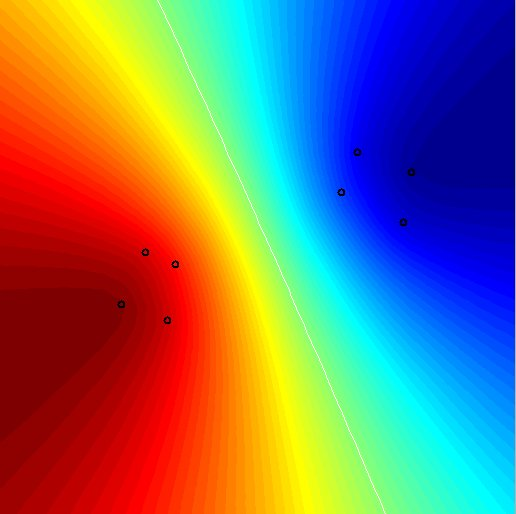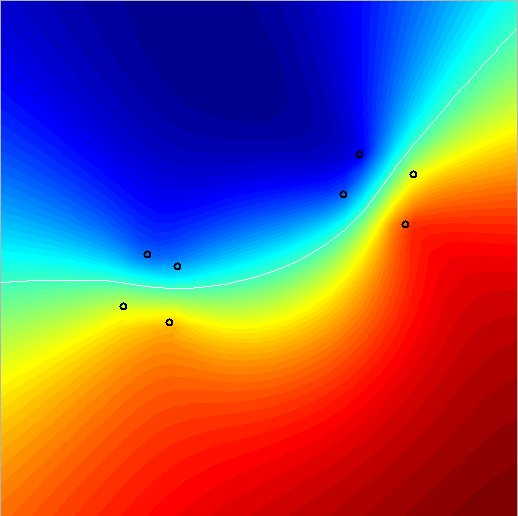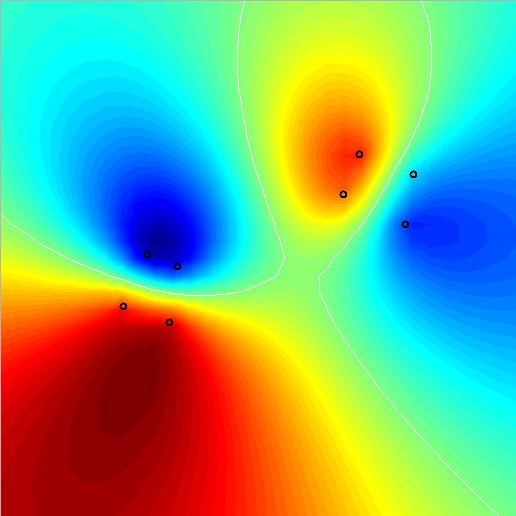```The usual PCA can only extract two linear features (= projections on the
main variance directions) on two-dimensional data. The kernel PCA is much
more powerful: For n data points, n features can be constructed, which are
arbitrary nonlinear or invariant, etc. depending on the chosen kernel. The
components are sorted with decreasing variance. The component on which to
project can be chosen by the "<<" and ">>" buttons and the edit field. For
indefinite kernels, the valuably negative variance components will be
located at the end of the spectrum.

6. Enclosing hypersphere:
```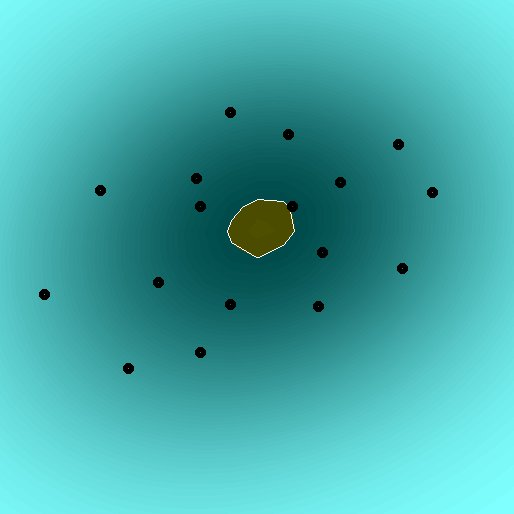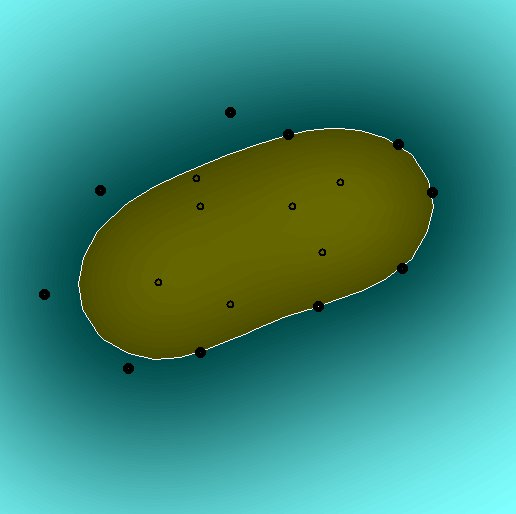```For clustering or one-class classification, an enclosing sphere can be
defined for given data. A kernelized version as given in Shawe-Taylor &
Cristianini is implemented here. The parameter "nu", which is related to
the ratio of support vectors and bounded support vectors must be chosen.

For details on these kernel methods, refer to

Schoelkopf, B. and Smola, A. Learning with Kernels: Support Vector
Machines, Regularization, Optimization and Beyond. MIT Press, 2002.

Shawe-Taylor, J. and Cristianini, N. Kernel Methods for Pattern Analysis.
Cambridge University Press, 2004.

Transformation:
---------------
```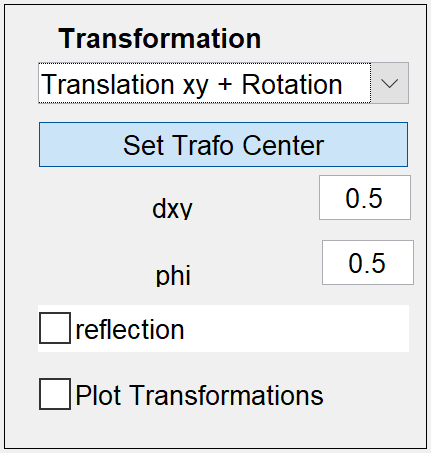```This field enables defining the pattern-variations, which should be
incorporated into kernels as invariances. This means, simple 2D-point
transformations are defined here, which define a set of "equivalent"
points for each point in the plane. A popupmenu enables to define
continuous transformations comprising
* simple translation in x, y and a combined x/y diagonal direction
* centered operations as rotation and scaling. The corresponding
reference point can be set by the appropriate button "Set Trafo Center"
* highly nonlinear transformation of shift along a sinusoidal curve.
* combined transformations: x/y-shift and rotation.
A checkbox "reflection" enables independent combination with a discrete
transformation, the reflection along a vertical axis, which is defined to
pass through the transformation center. The checkbox "Plot
Transformations" overlays the kernel plot with the transformations of all
points.

Invariant Kernel:
-----------------
``````Here the type of invariant kernel can be set.

1. Transformation-Integration Kernels (TI-Kernels):
```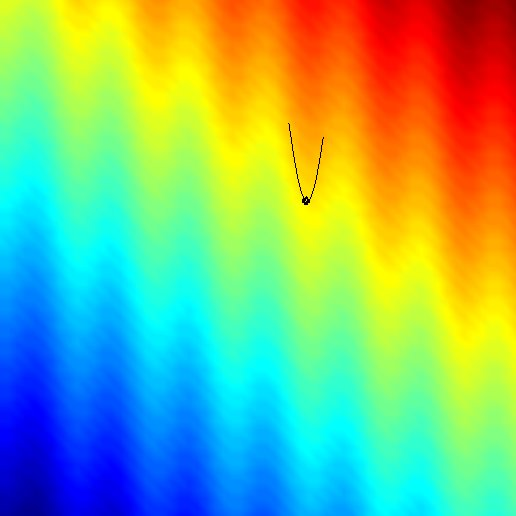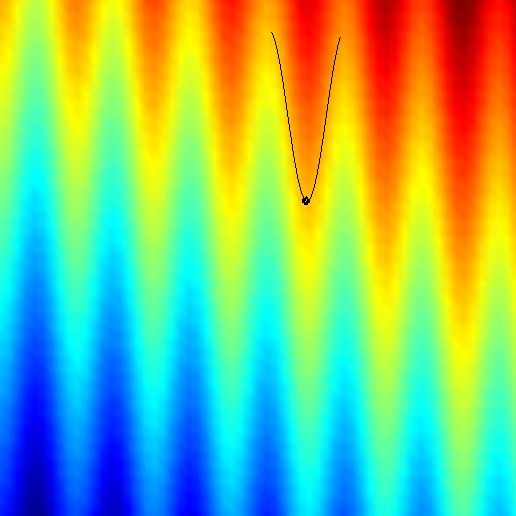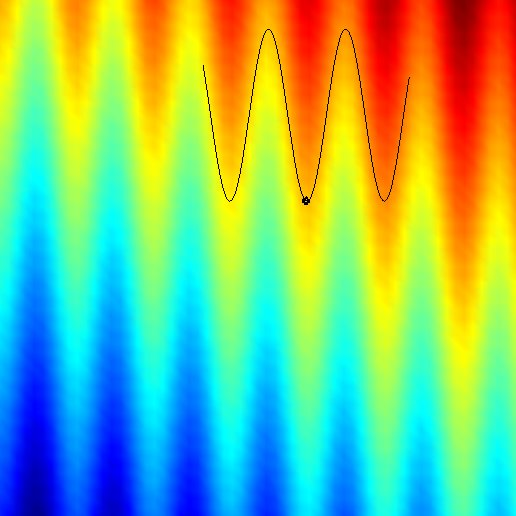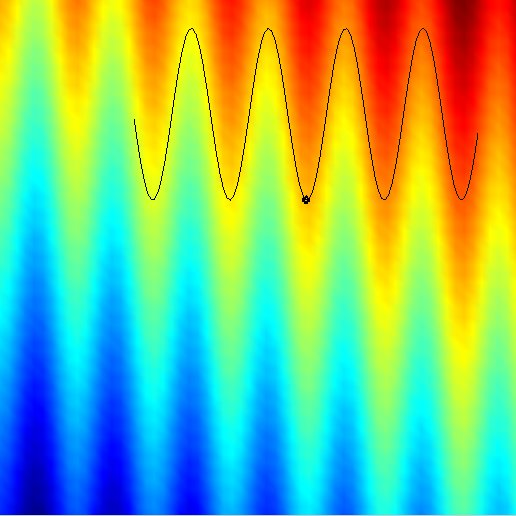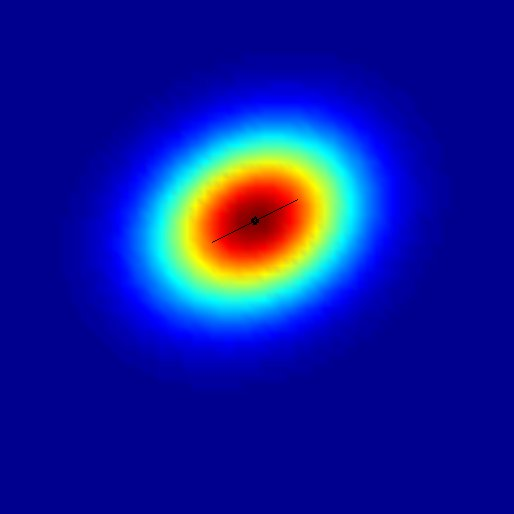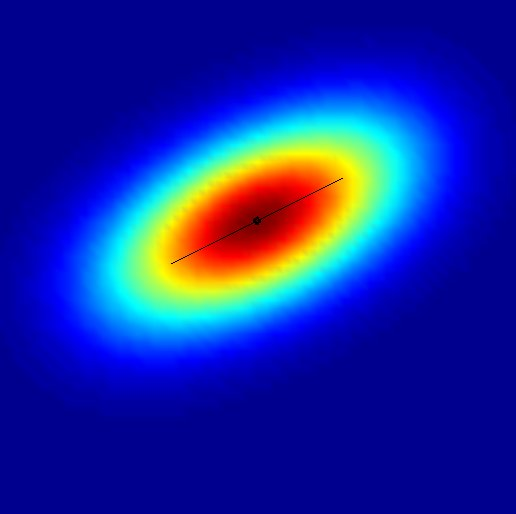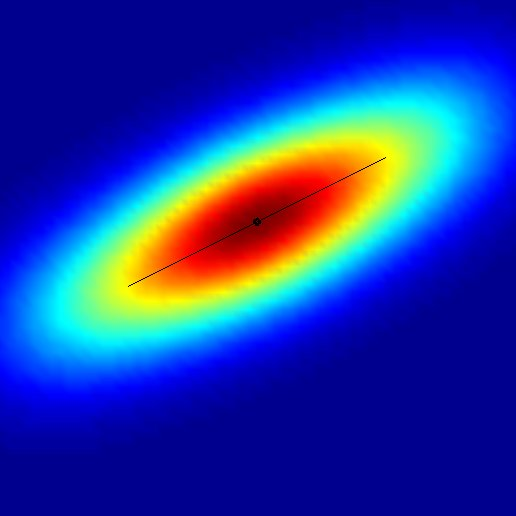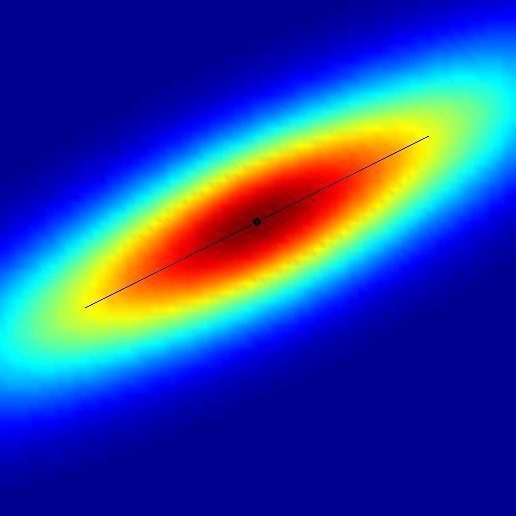```The technique of integration over transformation groups or subsets thereof
leads to the TI-kernels. In general the kernels are defined for infinite
sets of transformations. However for computational feasibility, the
kernels here are computed by finite sampling of the transformation sets.
The number of trafo-evaluations can be adjusted by "trafo-evals". More
details on these kernels can be found in

Haasdonk, B., Vossen, A. and Burkhardt, H., Invariance in Kernel Methods
by Haar-Integration Kernels.  SCIA 2005, Scandinavian Conference on Image
Analysis, pp. 841-851, Springer-Verlag, 2005.

2. Invariant Distance Substitution Kernels (IDS-Kernels):
```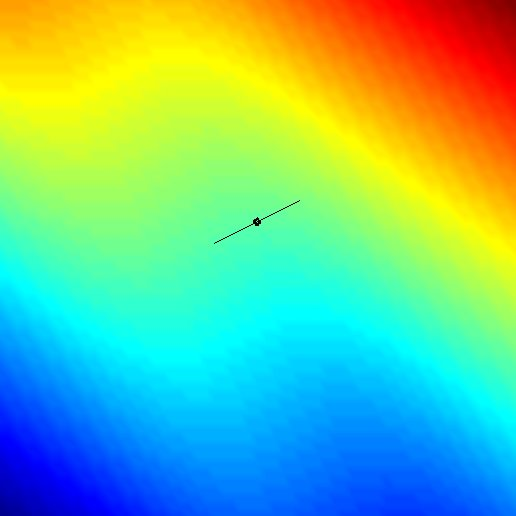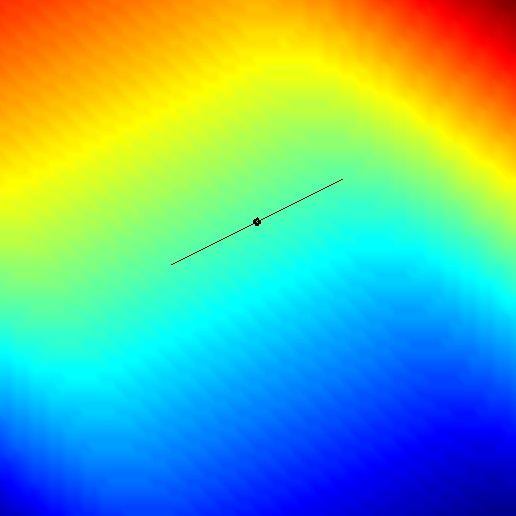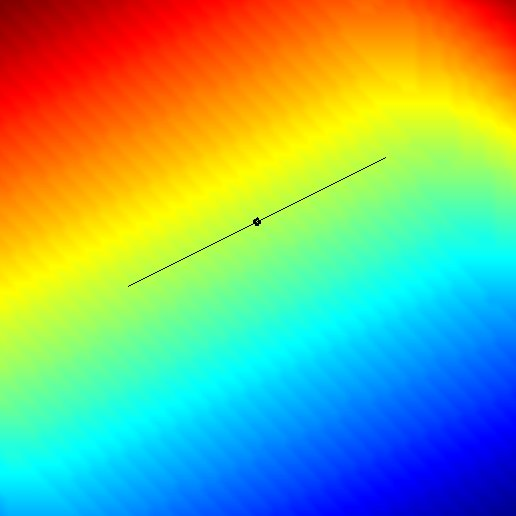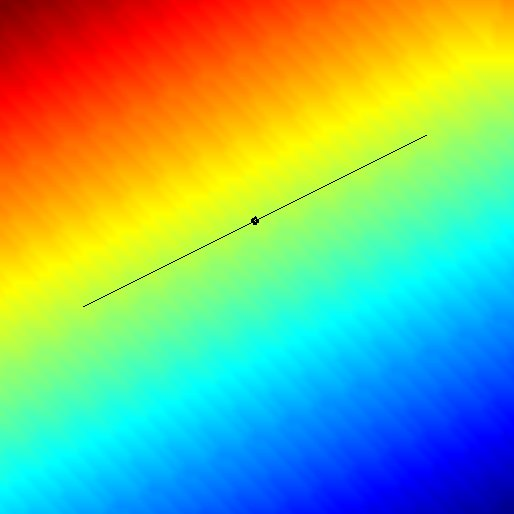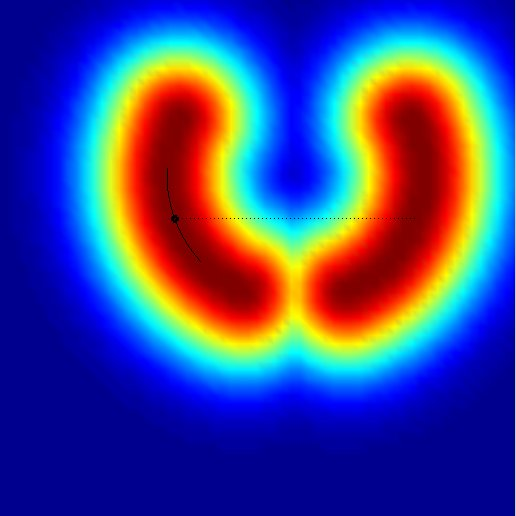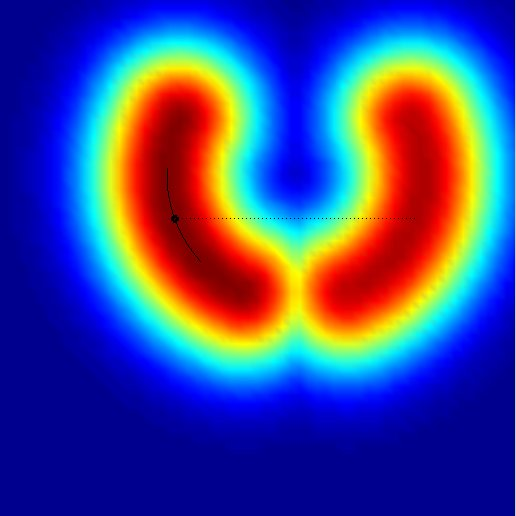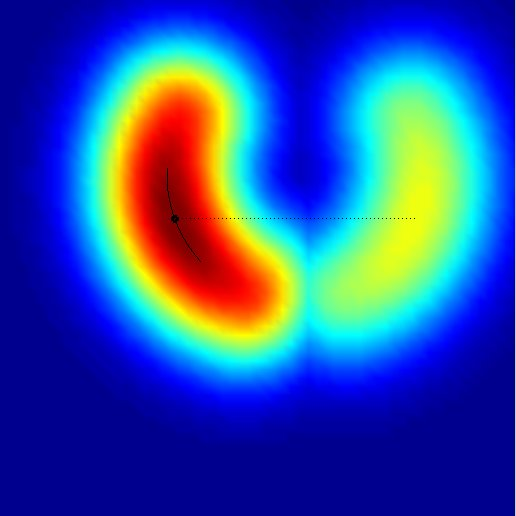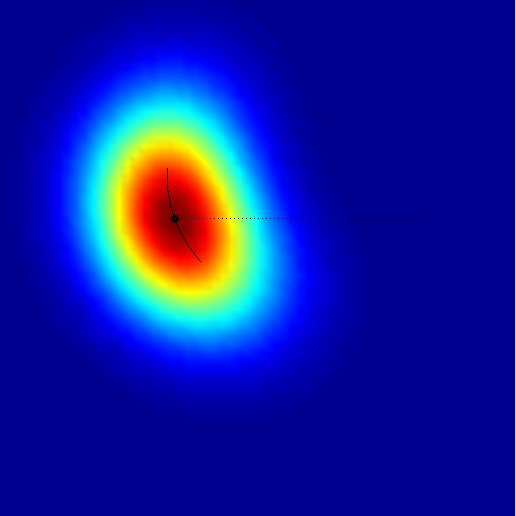```By computing the distance between the sets of transformed patterns, these
distances can be substituted in ordinary distance- and inner-product-based
kernels. As with the HI-kernels, the sampling of the transformation sets
can be specified. Additionally, a "origin" must be chosen in case of
inner-product base-kernels. If no precise invariance is wanted, the
parameter "Regularization lambda" interpolates between the precise
invariant case (lambda=0) and the ordinary non-invariant base-kernel
(lambda=Inf). More on these kernels can be found in

Haasdonk, B. and Burkhardt, H. Invariant Kernels for Pattern Analysis
and Machine Learning.
Machine Learning, 68:35-61, 2007.

3. Tangent Distance Kernels (TD-Kernels):
```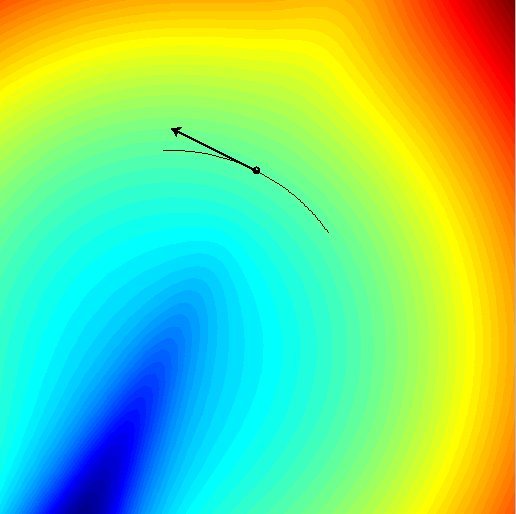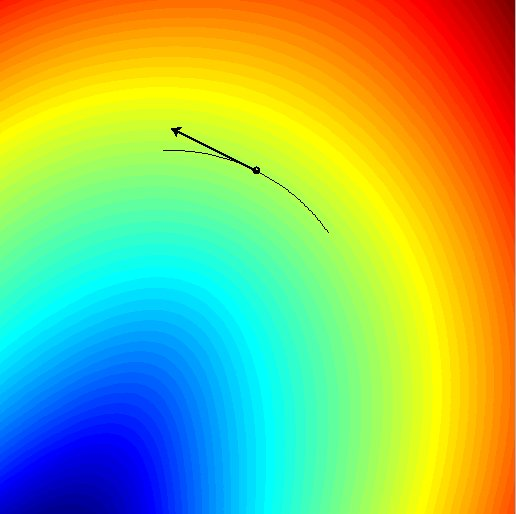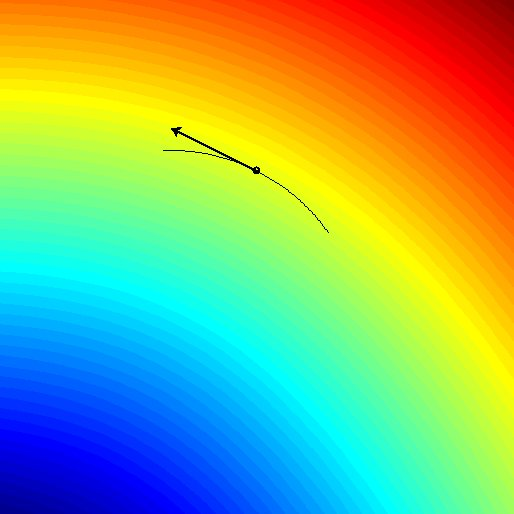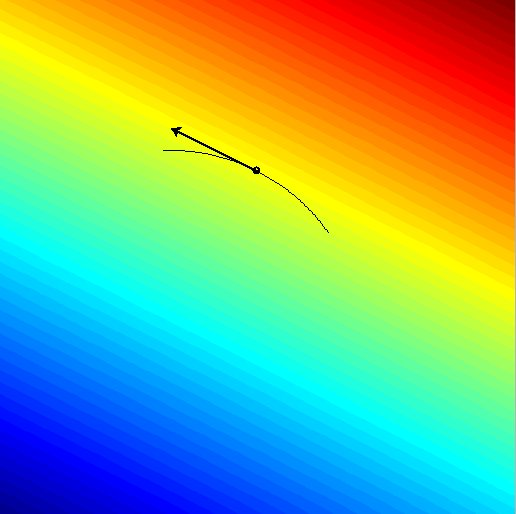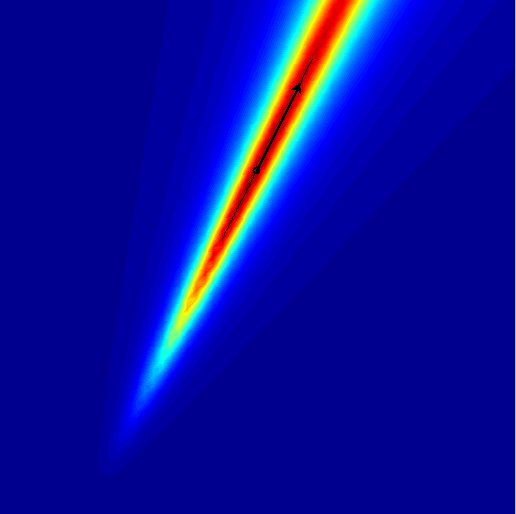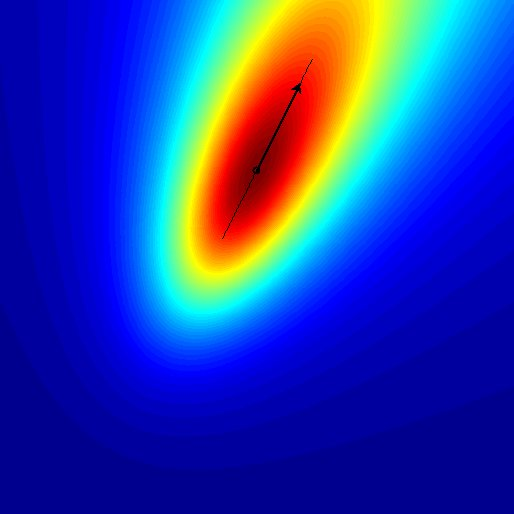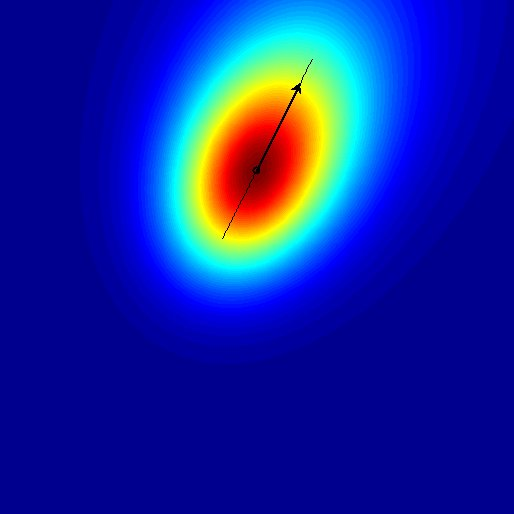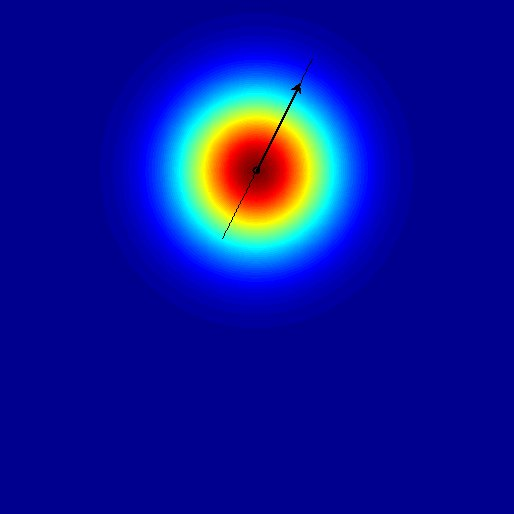```By approximating the continuous transformation manifolds (so discrete
transformations are excluded here) with linear spaces, the distance
computation can be performed very efficiently. Again the inner-product
based kernels require a choice of an "DS Origin" for the distance
substitution. Again the invariance degree can be adjusted by a
regularization parameter. 3 different symmetric versions of tangent
distance are implemented, the two-sided (2S), the mean of two one-sided
(MN) and the midpoint tangent distance (MP). Additionally the
non-symmetric one-sided distance is available, which however has to be
taken with care in kernel methods, as the kernel matrices are not
symmetric. The tangents can be "normalized" before tangent distance
computation, and plotting of the tangents can be switched on/off by "Plot
Tangents". More on these kernels can be found in

Haasdonk, B., Keysers, D., Tangent Distance Kernels for Support Vector
Machines.  ICPR 2002, International Conference on Pattern Recognition,
IEEE, 2002.

(C) Bernard Haasdonk, 2005-2019

```